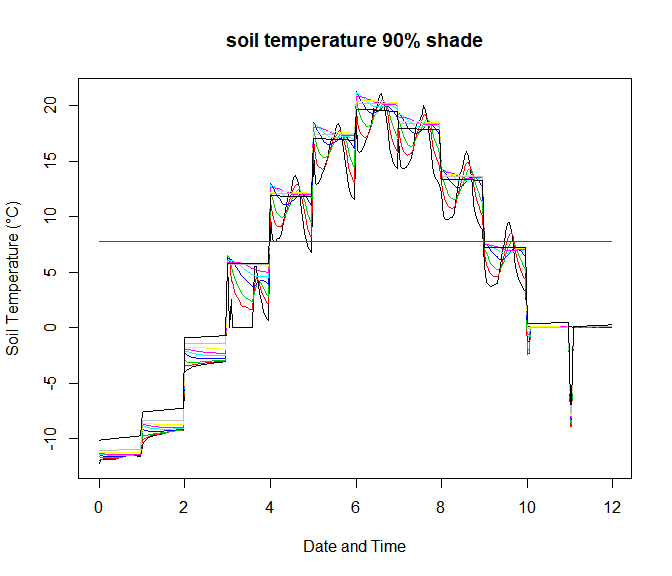# Monthly example of inputs and outputs of the NicheMapR microclimate model

#### 2019-10-08

This vignette runs through the core inputs and outputs of the NicheMapR microclimate model, using monthly mean inputs for Madison Wisconsin, USA. It provides a basis for developing scripts and functions that are customised to particular databases of climate/weather, terrain, vegetation and soil properties. See also the microclimate-hourly-input-example vignette for an illustration of how to set the model to run from hourly input across all days of a year. It is Appendix 3 of the NicheMapR microclimate software note, Porter and Kearney (2017). NicheMapR - an R package for biophysical modelling: the microclimate model. Ecography 40(5) p. 664-674 DOI 10.1111/ecog.02360.

### Model mode settings

library(NicheMapR) # load the NicheMapR package

writecsv <- 0 # make Fortran program write output as csv files
microdaily <- 0 # run microclimate model as normal, where each day is iterated 3 times starting with the initial condition of uniform soil temp at mean monthly temperature
runshade <- 1 # run the model twice, once for each shade level (1) or just for the first shade level (0)?
runmoist <- 1 # run soil moisture model (0=no, 1=yes)?
snowmodel <- 1 # run the snow model (0=no, 1=yes)? - note that this runs slower
hourly <- 0 # run from hourly weather input (0=no, 1=yes)?
rainhourly <- 0 # run from hourly weather input (0=no, 1=yes)?
IR <- 0 # compute clear-sky longwave radiation using Campbell and Norman (1998) eq. 10.10 (includes humidity)
message <- 0 # do not allow the Fortran integrator to output warnings
fail <- 24 * 365 # how many restarts of the integrator before the Fortran program quits (avoids endless loops when solutions can't be found)

### Time and location parameters

doynum <- 12 # number of time intervals to generate predictions for over a year (must be 12 <= x <=365)
doy<-c(15, 46, 74, 105, 135, 166, 196, 227, 258, 288, 319, 349) # middle day of each month
idayst <- 1 # start month
ida <- 12 # end month
HEMIS <- 1 # chose hemisphere
ALAT <- 43 # degrees latitude
AMINUT <- 4.383 # minutes latitude
ALONG <- 89 # degrees longitude
ALMINT <- 24.074 # minutes latitude
ALREF <- 89 # reference longitude for time zone
EC <- 0.0167238 # Eccenricity of the earth's orbit (current value 0.0167238, ranges between 0.0034 to 0.058)

### Air and wind vertical profile parameters

RUF <- 0.004 # Roughness height (m), , e.g. sand is 0.05, grass may be 2.0, current allowed range: 0.001 (snow) - 2.0 cm.
Refhyt <- 2 # Reference height (m), reference height at which air temperature, wind speed and relative humidity input data are measured
Usrhyt <- 0.01# local height (m) at which air temperature, relative humidity and wind speed calculatinos will be made
ZH <- 0 # heat transfer roughness height (m) for Campbell and Norman air temperature/wind speed profile (invoked if greater than 1, 0.02 * canopy height in m if unknown)
D0 <- 0 # zero plane displacement correction factor (m) for Campbell and Norman air temperature/wind speed profile (0.6 * canopy height in m if unknown)
# Next four parameters are segmented velocity profiles due to bushes, rocks etc. on the surface
#IF NO EXPERIMENTAL WIND PROFILE DATA SET ALL THESE TO ZERO! (then roughness height is based on the parameter RUF)
Z01 <- 0 # Top (1st) segment roughness height(m)
Z02 <- 0 # 2nd segment roughness height(m)
ZH1 <- 0 # Top of (1st) segment, height above surface(m)
ZH2 <- 0 # 2nd segment, height above surface(m)

### Terrain and shading parameters

ALTT <- 226 # altitude (m)
slope <- 0 # slope (degrees, range 0-90)
azmuth <- 180 # aspect (degrees, 0 = North, range 0-360)
hori <- rep(0, 24) # enter the horizon angles (degrees) so that they go from 0 degrees azimuth (north) clockwise in 15 degree intervals
VIEWF <- 1 - sum(sin(hori * pi / 180)) / length(hori) # convert horizon angles to radians and calc view factor(s)
solonly <- 0 # Only run SOLRAD to get solar radiation? 1=yes, 0=no
lamb <- 0 # Return wavelength-specific solar radiation output?
IUV <- 0 # Use gamma function for scattered solar radiation? (computationally intensive)
minshade <- 0 # minimum available shade (%)
maxshade <- 90 # maximum available shade (%)
PCTWET <- 0 # percentage of surface area acting as a free water surface (%)

### Soil profile settings

DEP <- c(0, 2.5, 5, 10, 15, 20, 30, 50, 100, 200) # Soil nodes (cm) - keep spacing close near the surface, last value is where it is assumed that the soil temperature is at the annual mean air temperature
ERR <- 1.5 # Integrator error for soil temperature calculations

### Time varying environmental data

TIMINS <- c(0, 0, 1, 1)   # time of minima for air temp, wind, humidity and cloud cover (h), air & wind mins relative to sunrise, humidity and cloud cover mins relative to solar noon
TIMAXS <- c(1, 1, 0, 0)   # time of maxima for air temp, wind, humidity and cloud cover (h), air temp & wind maxs relative to solar noon, humidity and cloud cover maxs relative to sunrise
TMINN <- c(-14.3, -12.1, -5.1, 1.2, 6.9, 12.3, 15.2, 13.6, 8.9, 3, -3.2, -10.6) # minimum air temperatures (°C)
TMAXX <- c(-3.2, 0.1, 6.8, 14.6, 21.3, 26.4, 29, 27.7, 23.3, 16.6, 7.8, -0.4) # maximum air temperatures (°C)
RHMINN <- c(50.2, 48.4, 48.7, 40.8, 40, 42.1, 45.5, 47.3, 47.6, 45, 51.3, 52.8) # min relative humidity (%)
RHMAXX <- c(100, 100, 100, 100, 100, 100, 100, 100, 100, 100, 100, 100) # max relative humidity (%)
WNMINN <- c(4.9, 4.8, 5.2, 5.3, 4.6, 4.3, 3.8, 3.7, 4, 4.6, 4.9, 4.8) # min wind speed (m/s)
WNMAXX <- c(4.9, 4.8, 5.2, 5.3, 4.6, 4.3, 3.8, 3.7, 4, 4.6, 4.9, 4.8) # max wind speed (m/s)
CCMINN <- c(50.3, 47, 48.2, 47.5, 40.9, 35.7, 34.1, 36.6, 42.6, 48.4, 61.1, 60.1) # min cloud cover (%)
CCMAXX <- c(50.3, 47, 48.2, 47.5, 40.9, 35.7, 34.1, 36.6, 42.6, 48.4, 61.1, 60.1) # max cloud cover (%)
RAINFALL <- c(28, 28.2, 54.6, 79.7, 81.3, 100.1, 101.3, 102.5, 89.7, 62.4, 54.9, 41.2) # monthly mean rainfall (mm)
TAIRhr <- rep(0, 24*doynum) # hourly air temperatures (°C), not used unless 'hourly=1'
RHhr <- rep(0, 24*doynum) # hourly relative humidity (%), not used unless 'hourly=1'
WNhr <- rep(0, 24*doynum) # hourly wind speed (m/s), not used unless 'hourly=1'
CLDhr <- rep(0, 24*doynum) # hourly cloud cover (%), not used unless 'hourly=1'
SOLRhr <- rep(0, 24*doynum) # hourly solar radiation (W/m2, not used unless 'hourly=1'
RAINhr <- rep(0, 24*doynum) # hourly rainfall (mm), not used unless 'hourly=1'
ZENhr <- rep(-1, 24*doynum) # hourly zenith angle (degrees), not used unless 'hourly=1'
tannul <- mean(c(TMAXX, TMINN)) # annual mean temperature for getting monthly deep soil temperature (°C)
tannulrun <- rep(tannul, doynum) # monthly deep soil temperature (2m) (°C)
SoilMoist <- c(0.42, 0.42, 0.42, 0.43, 0.44, 0.44, 0.43, 0.42, 0.41, 0.42, 0.42, 0.43) # soil moisture (decimal %, 1 means saturated)
# creating the arrays of environmental variables that are assumed not to change with month for this simulation
MAXSHADES <- rep(maxshade, doynum) # daily max shade (%)
MINSHADES <- rep(minshade, doynum) # daily min shade (%)
SLES <- rep(SLE, doynum) # set up vector of ground emissivities for each day
REFLS <- rep(REFL, doynum) # set up vector of soil reflectances for each day
PCTWET <- rep(PCTWET, doynum) # set up vector of soil wetness for each day

### Soil properties

# set up a profile of soil properites with depth for each day to be run
Numtyps <- 1 # number of soil types
Nodes <- matrix(data = 0, nrow = 10, ncol = doynum) # array of all possible soil nodes
Nodes[1, 1:doynum] <- 10 # deepest node for first substrate type

# soil thermal parameters
Thcond <- 1.25 # soil minerals thermal conductivity (W/mC)
Density <- 2.560 # soil minerals density (Mg/m3)
SpecHeat <- 870 # soil minerals specific heat (J/kg-K)
BulkDensity <- 2.56 # soil bulk density (Mg/m3)
SatWater <- 0.26 # volumetric water content at saturation (0.1 bar matric potential) (m3/m3)
#SoilMoist<-rep(SoilMoist,timeinterval) # soil moisture

# now make the depth-specific soil properties matrix
# columns are:
#1) bulk density (Mg/m3)
#2) volumetric water content at saturation (0.1 bar matric potential) (m3/m3)
#3) thermal conductivity (W/mK)
#4) specific heat capacity (J/kg-K)
#5) mineral density (Mg/m3)
soilprops<-matrix(data = 0, nrow = 10, ncol = 5) # create an empty soil properties matrix
soilprops[1, 1]<-BulkDensity # insert soil bulk density to profile 1
soilprops[1, 2]<-SatWater # insert saturated water content to profile 1
soilprops[1, 3]<-Thcond # insert thermal conductivity to profile 1
soilprops[1, 4]<-SpecHeat # insert specific heat to profile 1
soilprops[1, 5]<-Density # insert mineral density to profile 1
soilinit<-rep(tannul, 20) # make inital soil temps equal to mean annual

### Soil moisture parameters

# note that these are set for sand (Table 9.1 in Campbell and Norman, 1995)
PE <- rep(0.7, 19) #air entry potential J/kg
KS <- rep(0.0058, 19) #saturated conductivity, kg s/m3
BB <- rep(1.7, 19) #soil 'b' parameter
BD <- rep(1.3, 19) # soil bulk density, Mg/m3
DD <- rep(Density, 19)
L <- c(0, 0, 8.2, 8.0, 7.8, 7.4, 7.1, 6.4, 5.8, 4.8, 4.0, 1.8, 0.9, 0.6, 0.8, 0.4 ,0.4, 0, 0) * 10000 # root density at each node, mm/m3 (from Campell 1985 Soil Physics with Basic, p. 131)
R1 <- 0.001 #root radius, m}\cr\cr
RW <- 2.5e+10 #resistance per unit length of root, m3 kg-1 s-1
RL <- 2e+6 #resistance per unit length of leaf, m3 kg-1 s-1
PC <- -1500 #critical leaf water potential for stomatal closure, J kg-1
SP <- 10 #stability parameter for stomatal closure equation, -
IM <- 1e-06 #maximum allowable mass balance error, kg
MAXCOUNT <- 500 #maximum iterations for mass balance, -
LAI <- rep(0.1, doynum) # leaf area index, used to partition traspiration/evaporation from PET
rainmult <- 1 # rainfall multiplier to impose catchment
maxpool <- 10 # max depth for water pooling on the surface, mm (to account for runoff)
evenrain <- 1 # spread daily rainfall evenly across 24hrs (1) or one event at midnight (2)
SoilMoist_Init <- rep(0.2, 10) # initial soil water content for each node, m3/m3
moists <- matrix(nrow = 10, ncol = doynum, data = 0) # set up an empty vector for soil moisture values through time
moists[1:10, ] <- SoilMoist_Init # insert inital soil moisture

### Snow model parameters

snowtemp <- 1.5 # temperature at which precipitation falls as snow (used for snow model)
snowdens <- 0.375 # snow density (mg/m3)
densfun <- c(0.5979, 0.2178, 0.001, 0.0038) # slope and intercept of linear model of snow density as a function of day of year - if it is c(0,0) then fixed density used
snowmelt <- 1 # proportion of calculated snowmelt that doesn't refreeze
undercatch <- 1 # undercatch multipier for converting rainfall to snow
rainmelt <- 0.0125 # parameter in equation from Anderson's SNOW-17 model that melts snow with rainfall as a function of air temp
snowcond <- 0 # effective snow thermal conductivity W/mC (if zero, uses inbuilt function of density)
intercept <- 0 # snow interception fraction for when there's shade (0-1)
grasshade <- 0 # if 1, means shade is removed when snow is present, because shade is cast by grass/low veg

### Intertidal simulation parameters (currently experimental and untested)

# intertidal simulation input vector (col 1 = tide in(1)/out(0), col 2 = sea water temperature in °C, col 3 = % wet from wave splash)
tides <- matrix(data = 0, nrow = 24 * doynum, ncol = 3) # matrix for tides

### Collating all input parameters

# input parameter vector
microinput<-c(doynum, RUF, ERR, Usrhyt, Refhyt, Numtyps, Z01, Z02, ZH1, ZH2, idayst, ida, HEMIS, ALAT, AMINUT, ALONG, ALMINT, ALREF, slope, azmuth, ALTT, CMH2O, microdaily, tannul, EC, VIEWF, snowtemp, snowdens, snowmelt, undercatch, rainmult, runshade, runmoist, maxpool, evenrain, snowmodel, rainmelt, writecsv, densfun, hourly, rainhourly, lamb, IUV, RW, PC, RL, SP, R1, IM, MAXCOUNT, IR, message, fail, snowcond, intercept, grasshade, solonly, ZH, D0)

# Final input list - all these variables are expected by the input argument of the Fortran microclimate subroutine
micro<-list(microinput = microinput, tides = tides, doy = doy, SLES = SLES, DEP = DEP, Nodes = Nodes, MAXSHADES = MAXSHADES, MINSHADES = MINSHADES, TIMAXS = TIMAXS, TIMINS = TIMINS, TMAXX = TMAXX, TMINN = TMINN, RHMAXX = RHMAXX, RHMINN = RHMINN, CCMAXX = CCMAXX, CCMINN = CCMINN, WNMAXX = WNMAXX, WNMINN = WNMINN, TAIRhr = TAIRhr, RHhr = RHhr, WNhr = WNhr, CLDhr = CLDhr, SOLRhr = SOLRhr, RAINhr = RAINhr, ZENhr = ZENhr, REFLS = REFLS, PCTWET = PCTWET, soilinit = soilinit, hori = hori, TAI = TAI, soilprops = soilprops, moists = moists, RAINFALL = RAINFALL, tannulrun = tannulrun, PE = PE, KS = KS, BB = BB, BD = BD, DD = DD, L = L, LAI = LAI)

### Executing the microclimate model

microut <- microclimate(micro) # run the model in Fortran

### Retrieving the data

metout <- as.data.frame(microut$metout[1:(doynum * 24), ]) # retrieve above ground microclimatic conditions, min shade shadmet <- as.data.frame(microut$shadmet[1:(doynum * 24), ]) # retrieve above ground microclimatic conditions, max shade
soil <- as.data.frame(microut$soil[1:(doynum * 24), ]) # retrieve soil temperatures, minimum shade shadsoil <- as.data.frame(microut$shadsoil[1:(doynum * 24), ]) # retrieve soil temperatures, maximum shade
soilmoist <- as.data.frame(microut$soilmoist[1:(doynum * 24), ]) # retrieve soil moisture, minimum shade shadmoist <- as.data.frame(microut$shadmoist[1:(doynum * 24), ]) # retrieve soil moisture, maximum shade
humid <- as.data.frame(microut$humid[1:(doynum * 24), ]) # retrieve soil humidity, minimum shade shadhumid <- as.data.frame(microut$shadhumid[1:(doynum * 24), ]) # retrieve soil humidity, maximum shade
soilpot <- as.data.frame(microut$soilpot[1:(doynum * 24), ]) # retrieve soil water potential, minimum shade shadpot <- as.data.frame(microut$shadpot[1:(doynum * 24), ]) # retrieve soil water potential, maximum shade

### Plotting the results

# append dates
days <- rep(seq(1, 12), 24)
days <- days[order(days)]
dates <- days + metout$TIME / 60 / 24 - 1 # dates for hourly output dates2 <- seq(1, 12, 1) # dates for daily output plotmetout <- cbind(dates, metout) plotsoil <- cbind(dates, soil) plotshadmet <- cbind(dates, shadmet) plotshadsoil <- cbind(dates, shadsoil) minshade <- micro$MINSHADES
maxshade <- micro\$MAXSHADES

# plotting above-ground conditions in minimum shade
with(plotmetout, {plot(TALOC ~ dates, xlab = "Date and Time", ylab = "Air Temperature (°C)", type = "l", main = paste("air temperature, ", minshade, "% shade", sep=""))})
with(plotmetout, {points(TAREF ~ dates, xlab = "Date and Time", ylab = "Air Temperature (°C)", type = "l", lty = 2, col = 'blue')})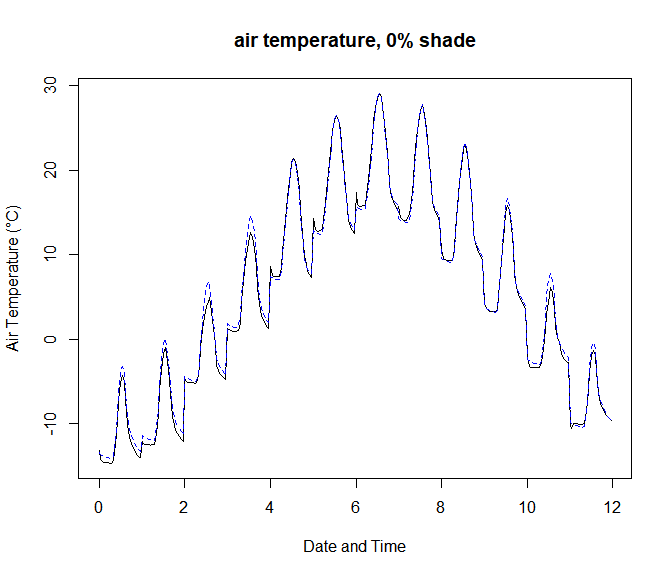with(plotmetout, {plot(RHLOC ~ dates, xlab = "Date and Time", ylab = "Relative Humidity (%)", type = "l", ylim = c(0, 100), main = paste("humidity, ", minshade, "% shade", sep = ""))})
with(plotmetout, {points(RH ~ dates, xlab = "Date and Time", ylab = "Relative Humidity (%)", type = "l", col = 'blue', lty = 2, ylim = c(0, 100))})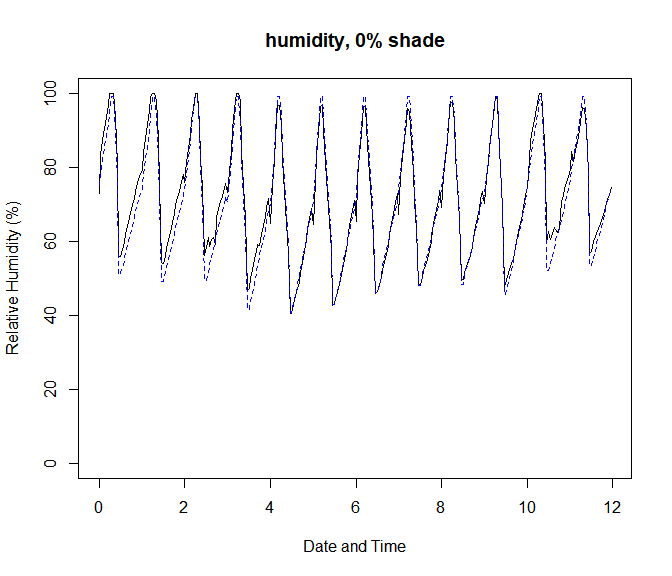with(plotmetout, {plot(TSKYC ~ dates, xlab = "Date and Time", ylab = "Sky Temperature (°C)", type = "l", main = paste("sky temperature, ", minshade, "% shade", sep = ""))})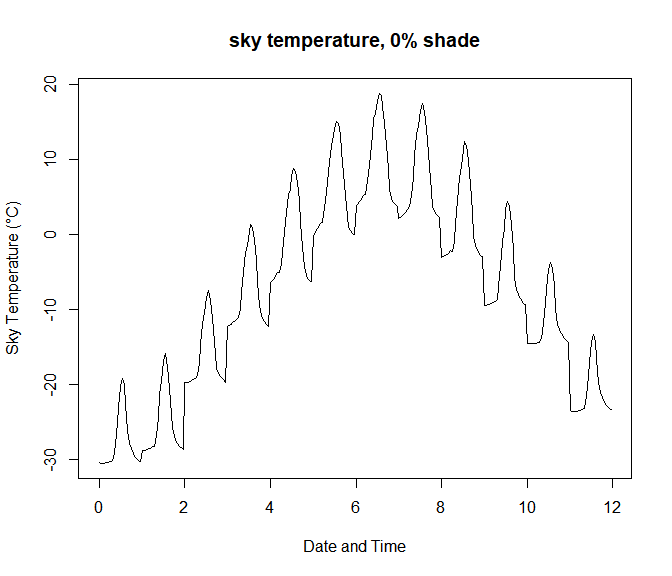with(plotmetout, {plot(VREF ~ dates, xlab = "Date and Time", ylab = "Wind Speed (m/s)"
, type = "l", main = "wind speed")})
with(plotmetout, {points(VLOC ~ dates, xlab = "Date and Time", ylab = "Wind Speed (m/s)", type = "l", lty = 2, col = 'blue')})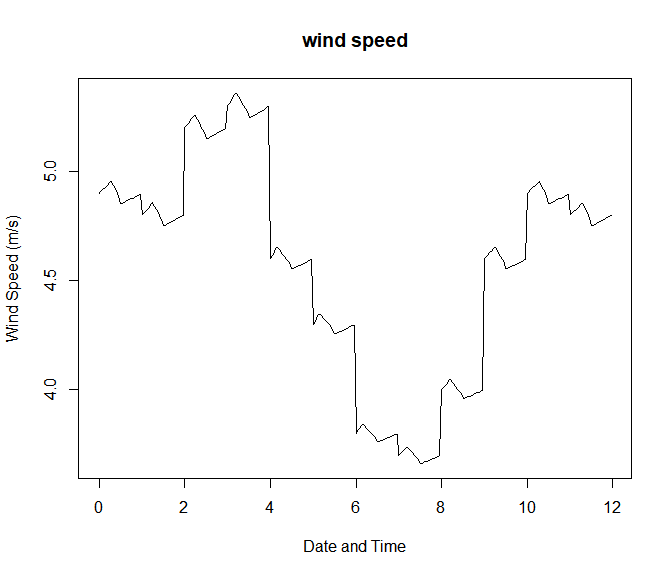with(plotmetout, {plot(ZEN ~ dates, xlab = "Date and Time", ylab = "Zenith Angle of Sun (deg)", type = "l", main = "solar angle, sun")})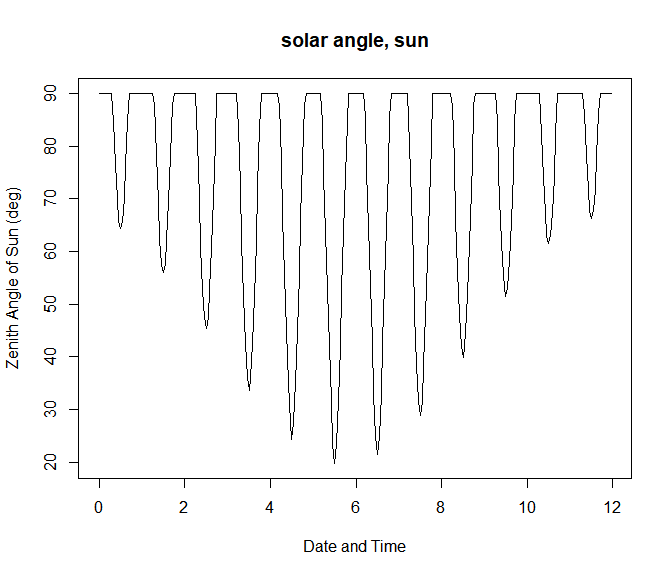with(plotmetout, {plot(SOLR ~ dates, xlab = "Date and Time", ylab = "Solar Radiation (W/m2)", type = "l", main = "solar radiation")})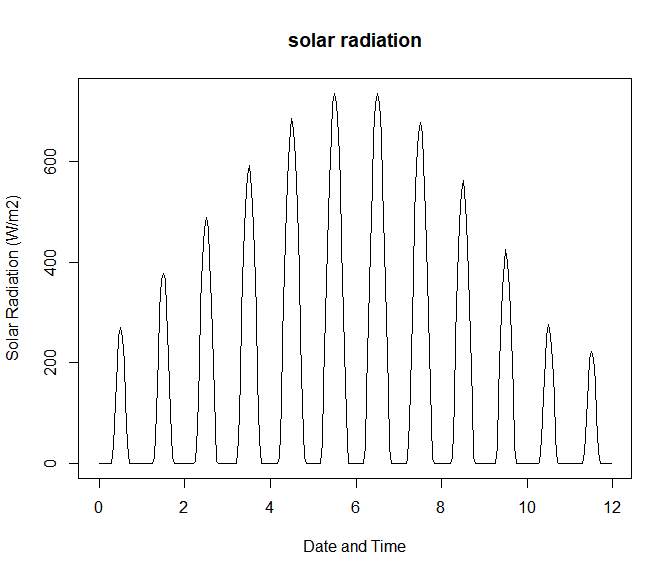# plotting soil temperature for minimum shade
for(i in 1:10){
if(i == 1){
plot(plotsoil[, i + 3] ~ plotsoil[, 1], xlab = "Date and Time", ylab = "Soil Temperature (°C)", col = i, type = "l", main = paste("soil temperature ", minshade, "% shade", sep=""))
}else{
points(plotsoil[, i + 3] ~ plotsoil[, 1], xlab = "Date and Time", ylab = "Soil Temperature (°C)", col = i, type = "l")
}
}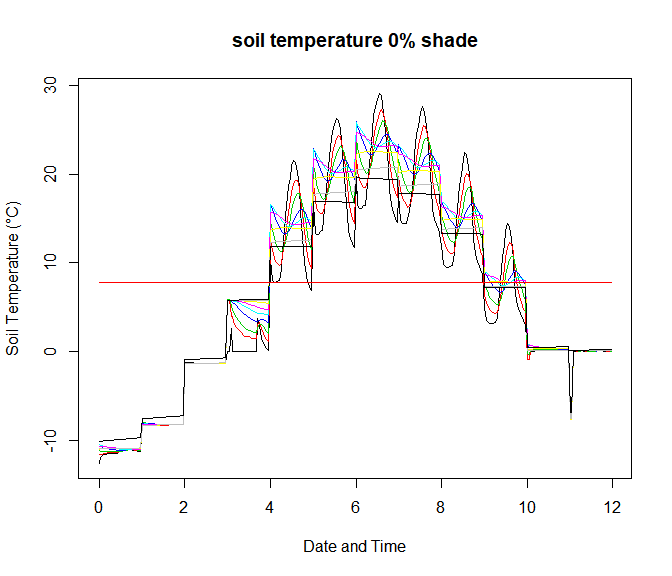# plotting above-ground conditions in maximum shade
with(plotshadmet, {plot(TALOC ~ dates, xlab = "Date and Time", ylab = "Air Temperature (°C)", type = "l", main = paste("air temperature, ", maxshade, "% shade", sep = ""))})
with(plotshadmet, {points(TAREF ~ dates, xlab = "Date and Time", ylab = "Air Temperature (°C)", type = "l", lty = 2, col = 'blue')})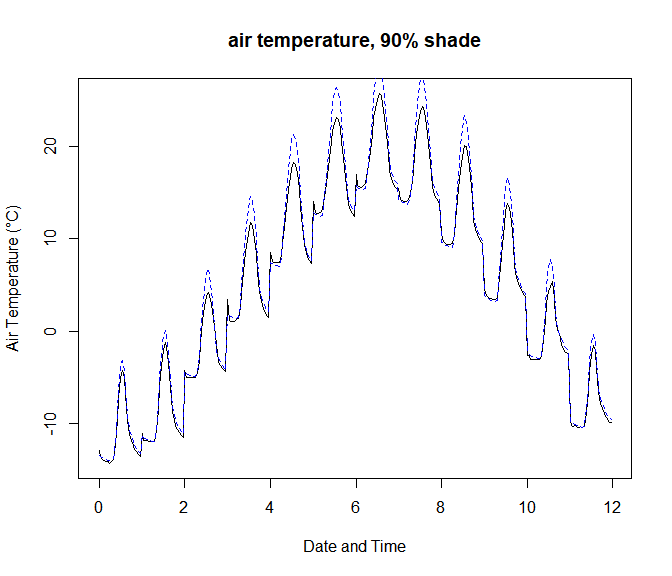with(plotshadmet,{plot(RHLOC ~ dates,xlab = "Date and Time", ylab = "Relative Humidity (%)"
, type = "l",ylim=c(0,100),main=paste("humidity, ",maxshade,"% shade",sep=""))})
with(plotshadmet, {points(RH ~ dates, xlab = "Date and Time", ylab = "Relative Humidity (%)", type = "l", col = 'blue', lty = 2, ylim = c(0, 100))})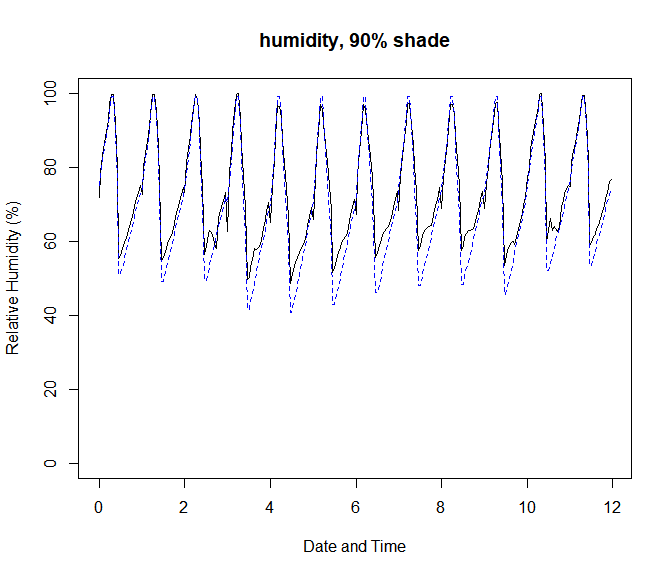with(plotshadmet, {plot(TSKYC ~ dates, xlab = "Date and Time", ylab = "Sky Temperature (°C)", type = "l", main = paste("sky temperature, ", maxshade, "% shade", sep = ""))})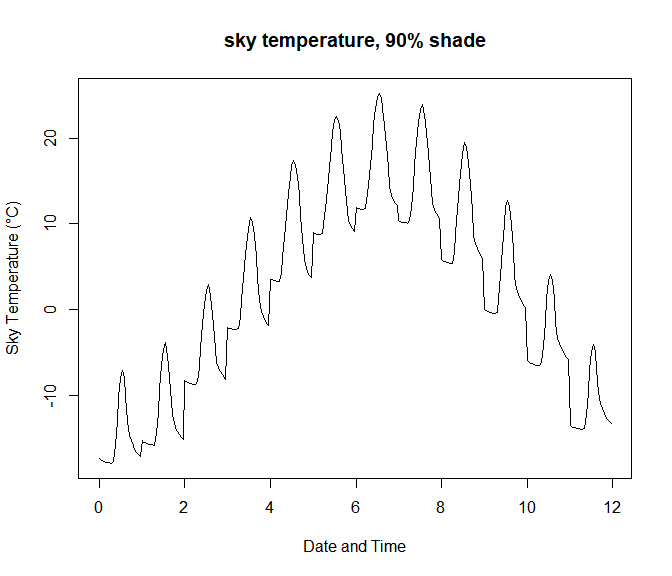# plotting soil temperature for maximum shade
for(i in 1:10){
if(i == 1){
plot(plotshadsoil[, i + 3] ~ plotshadsoil[, 1], xlab = "Date and Time", ylab = "Soil Temperature (°C)", col = i, type = "l", main = paste("soil temperature ", maxshade, "% shade", sep = ""))
}else{
points(plotshadsoil[, i + 3] ~ plotshadsoil[, 1], xlab = "Date and Time", ylab = "Soil Temperature (°C)", col = i, type = "l")
}
}